# Factoring PolynomialsPage 2

#### WATCH ALL SLIDES

Find the GCF of the following list of terms.

a3b2, a2b5 and a4b7

a3b2 = a · a · a · b · b

a2b5 = a · a · b · b · b · b · b

a4b7 = a · a · a · a · b · b · b · b · b · b · b

So the GCF is a · a · b · b = a2b2

Notice that the GCF of terms containing variables will use the smallest exponent found amongst the individual terms for each variable.

Greatest Common Factor

Example

Slide 10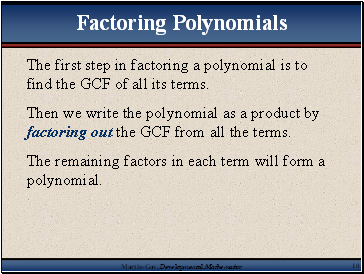## Factoring Polynomials

The first step in factoring a polynomial is to find the GCF of all its terms.

Then we write the polynomial as a product by factoring out the GCF from all the terms.

The remaining factors in each term will form a polynomial.

Slide 11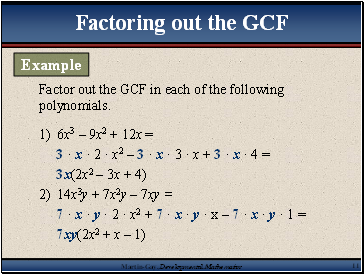## Factoring out the GCF

Factor out the GCF in each of the following polynomials.

1) 6x3 – 9x2 + 12x =

3 · x · 2 · x2 – 3 · x · 3 · x + 3 · x · 4 =

3x(2x2 – 3x + 4)

2) 14x3y + 7x2y – 7xy =

7 · x · y · 2 · x2 + 7 · x · y · x – 7 · x · y · 1 =

7xy(2x2 + x – 1)

Example

Slide 12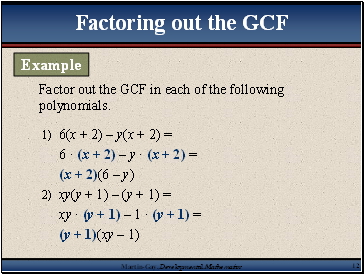Factor out the GCF in each of the following polynomials.

1) 6(x + 2) – y(x + 2) =

6 · (x + 2) – y · (x + 2) =

(x + 2)(6 – y)

2) xy(y + 1) – (y + 1) =

xy · (y + 1) – 1 · (y + 1) =

(y + 1)(xy – 1)

Factoring out the GCF

Example

Slide 13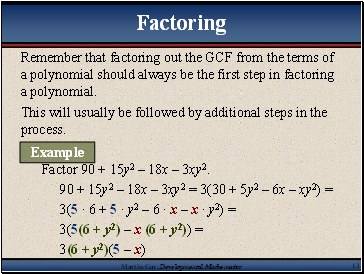## Factoring

Remember that factoring out the GCF from the terms of a polynomial should always be the first step in factoring a polynomial.

This will usually be followed by additional steps in the process.

Factor 90 + 15y2 – 18x – 3xy2.

90 + 15y2 – 18x – 3xy2 = 3(30 + 5y2 – 6x – xy2) =

3(5 · 6 + 5 · y2 – 6 · x – x · y2) =

3(5(6 + y2) – x (6 + y2)) =

3(6 + y2)(5 – x)

Example

Slide 14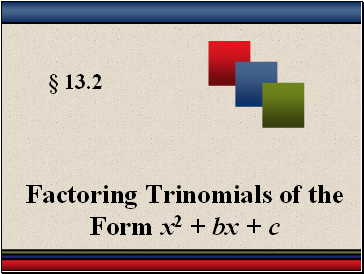§ 13.2

Factoring Trinomials of the Form x2 + bx + c

Slide 15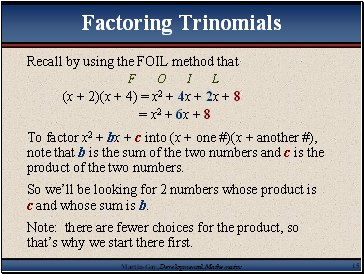## Factoring Trinomials

Recall by using the FOIL method that

F O I L

(x + 2)(x + 4) = x2 + 4x + 2x + 8

= x2 + 6x + 8

To factor x2 + bx + c into (x + one #)(x + another #), note that b is the sum of the two numbers and c is the product of the two numbers.

So we’ll be looking for 2 numbers whose product is c and whose sum is b.

Note: there are fewer choices for the product, so that’s why we start there first.

Slide 16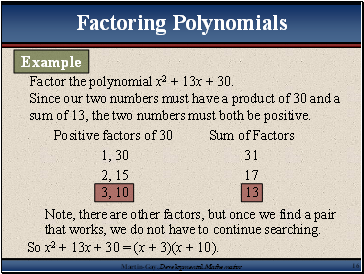## Factoring Polynomials

Factor the polynomial x2 + 13x + 30.

Since our two numbers must have a product of 30 and a sum of 13, the two numbers must both be positive.

Go to page:
1  2  3  4  5  6  7  8  9  10